Скачать презентацию Ontologies a system of concepts and relationships

0f3191b6272333a42b692241b796dcf4.ppt

• Количество слайдов: 15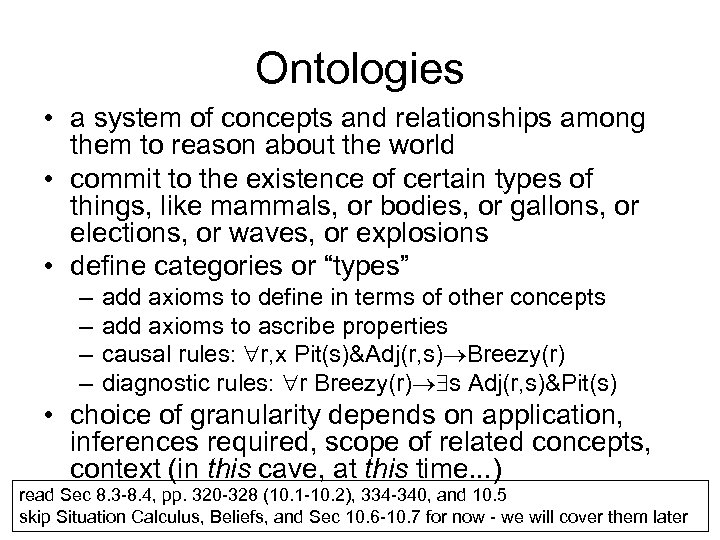Ontologies • a system of concepts and relationships among them to reason about the world • commit to the existence of certain types of things, like mammals, or bodies, or gallons, or elections, or waves, or explosions • define categories or “types” – – add axioms to define in terms of other concepts add axioms to ascribe properties causal rules: r, x Pit(s)&Adj(r, s) Breezy(r) diagnostic rules: r Breezy(r) s Adj(r, s)&Pit(s) • choice of granularity depends on application, inferences required, scope of related concepts, context (in this cave, at this time. . . ) read Sec 8. 3 -8. 4, pp. 320 -328 (10. 1 -10. 2), 334 -340, and 10. 5 skip Situation Calculus, Beliefs, and Sec 10. 6 -10. 7 for now - we will cover them later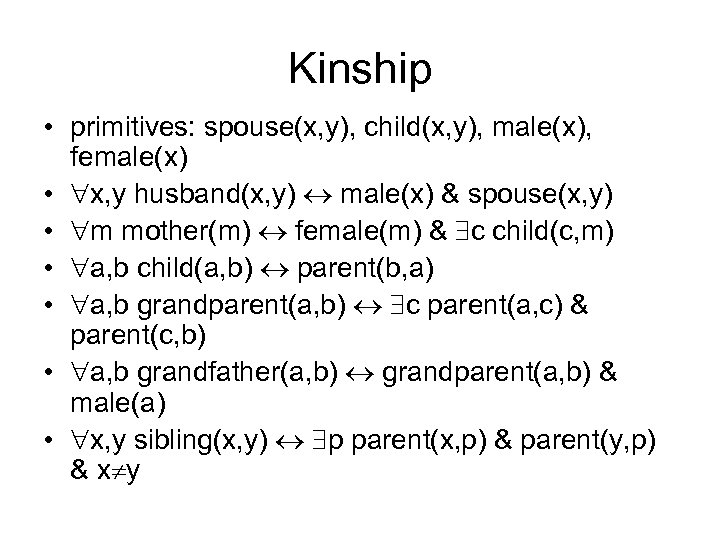Kinship • primitives: spouse(x, y), child(x, y), male(x), female(x) • x, y husband(x, y) male(x) & spouse(x, y) • m mother(m) female(m) & c child(c, m) • a, b child(a, b) parent(b, a) • a, b grandparent(a, b) c parent(a, c) & parent(c, b) • a, b grandfather(a, b) grandparent(a, b) & male(a) • x, y sibling(x, y) p parent(x, p) & parent(y, p) & x y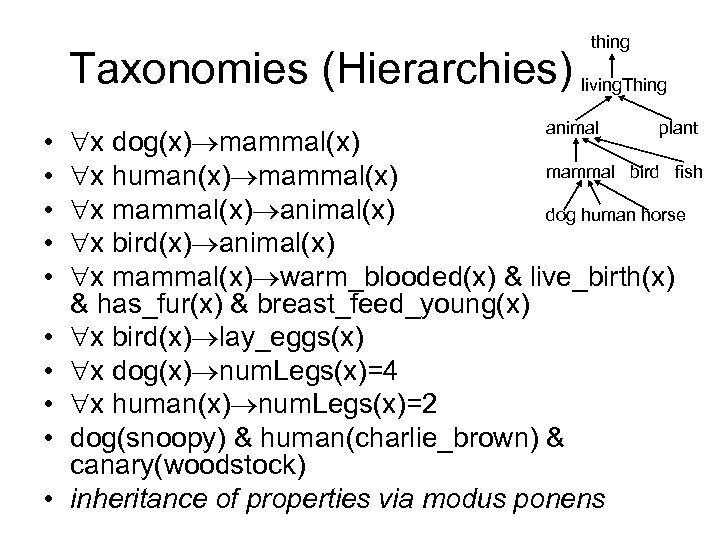thing Taxonomies (Hierarchies) living. Thing • • • animal plant x dog(x) mammal bird fish x human(x) mammal(x) x mammal(x) animal(x) dog human horse x bird(x) animal(x) x mammal(x) warm_blooded(x) & live_birth(x) & has_fur(x) & breast_feed_young(x) x bird(x) lay_eggs(x) x dog(x) num. Legs(x)=4 x human(x) num. Legs(x)=2 dog(snoopy) & human(charlie_brown) & canary(woodstock) inheritance of properties via modus ponens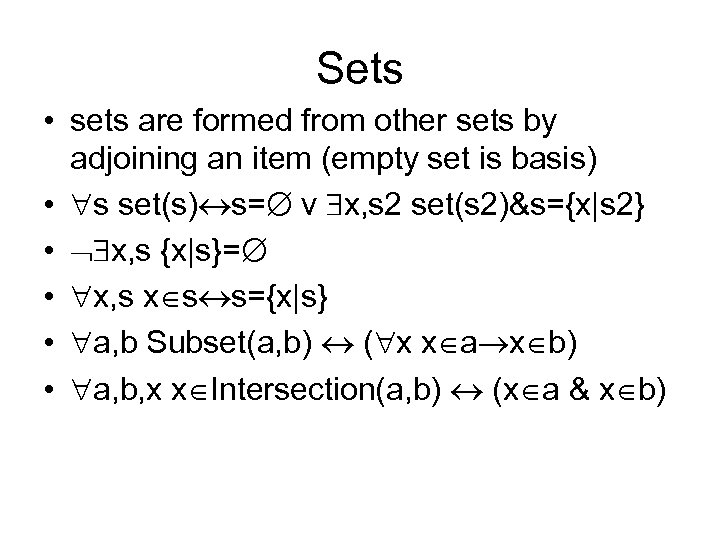Sets • sets are formed from other sets by adjoining an item (empty set is basis) • s set(s) s= v x, s 2 set(s 2)&s={x|s 2} • x, s {x|s}= • x, s x s s={x|s} • a, b Subset(a, b) ( x x a x b) • a, b, x x Intersection(a, b) (x a & x b)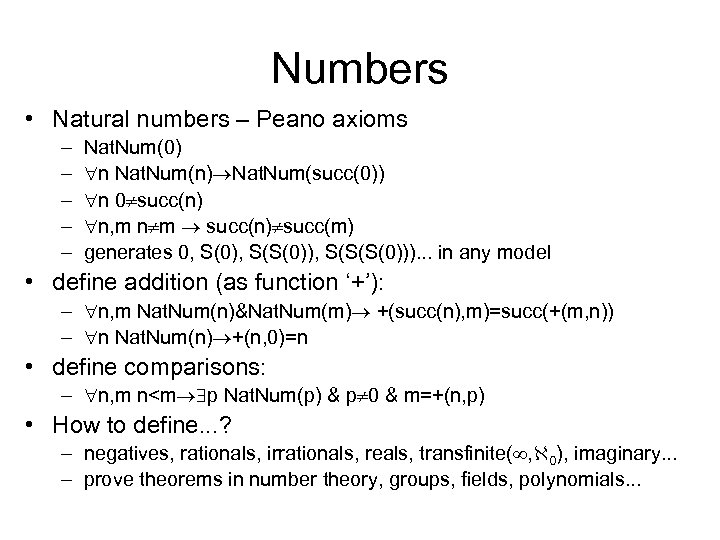Numbers • Natural numbers – Peano axioms – – – Nat. Num(0) n Nat. Num(n) Nat. Num(succ(0)) n 0 succ(n) n, m n m succ(n) succ(m) generates 0, S(0), S(S(0)), S(S(S(0))). . . in any model • define addition (as function ‘+’): – n, m Nat. Num(n)&Nat. Num(m) +(succ(n), m)=succ(+(m, n)) – n Nat. Num(n) +(n, 0)=n • define comparisons: – n, m n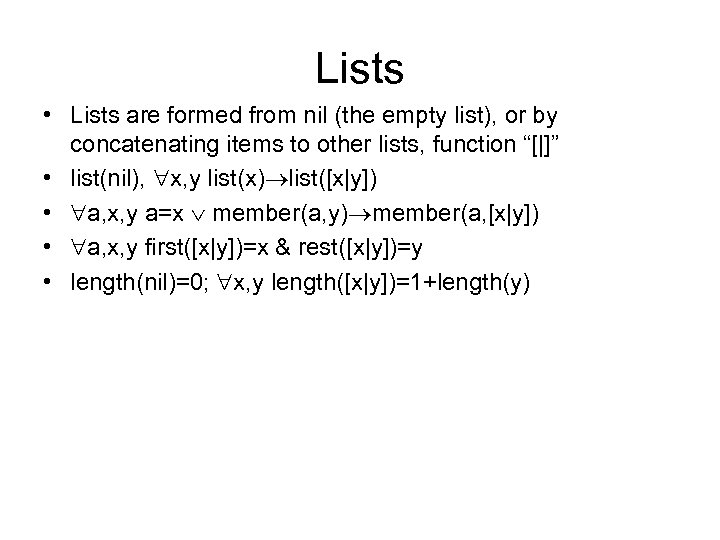Lists • Lists are formed from nil (the empty list), or by concatenating items to other lists, function “[|]” • list(nil), x, y list(x) list([x|y]) • a, x, y a=x member(a, y) member(a, [x|y]) • a, x, y first([x|y])=x & rest([x|y])=y • length(nil)=0; x, y length([x|y])=1+length(y)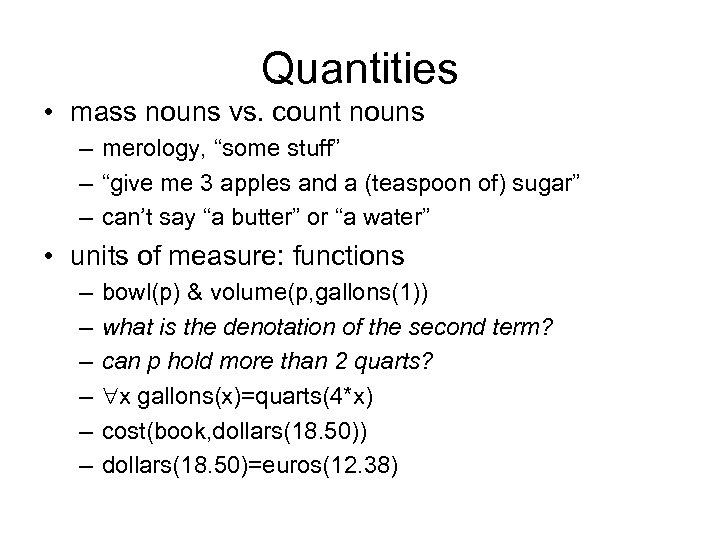Quantities • mass nouns vs. count nouns – merology, “some stuff” – “give me 3 apples and a (teaspoon of) sugar” – can’t say “a butter” or “a water” • units of measure: functions – – – bowl(p) & volume(p, gallons(1)) what is the denotation of the second term? can p hold more than 2 quarts? x gallons(x)=quarts(4*x) cost(book, dollars(18. 50)) dollars(18. 50)=euros(12. 38)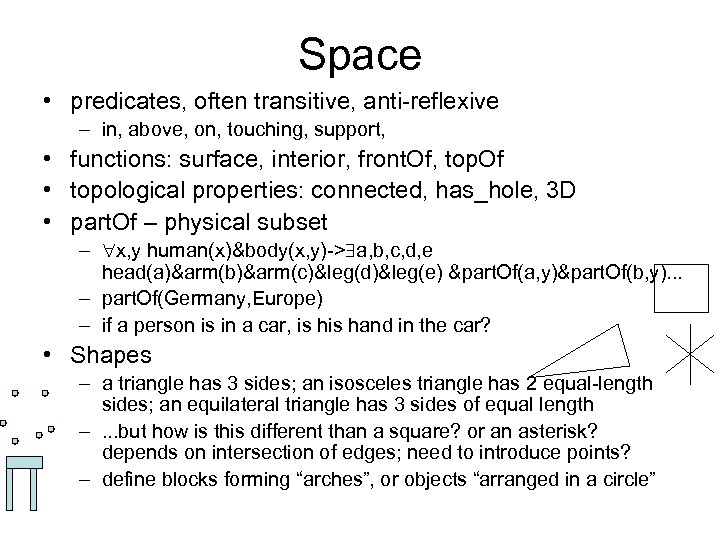Space • predicates, often transitive, anti-reflexive – in, above, on, touching, support, • functions: surface, interior, front. Of, top. Of • topological properties: connected, has_hole, 3 D • part. Of – physical subset – x, y human(x)&body(x, y)-> a, b, c, d, e head(a)&arm(b)&arm(c)&leg(d)&leg(e) &part. Of(a, y)&part. Of(b, y). . . – part. Of(Germany, Europe) – if a person is in a car, is hand in the car? • Shapes – a triangle has 3 sides; an isosceles triangle has 2 equal-length sides; an equilateral triangle has 3 sides of equal length –. . . but how is this different than a square? or an asterisk? depends on intersection of edges; need to introduce points? – define blocks forming “arches”, or objects “arranged in a circle”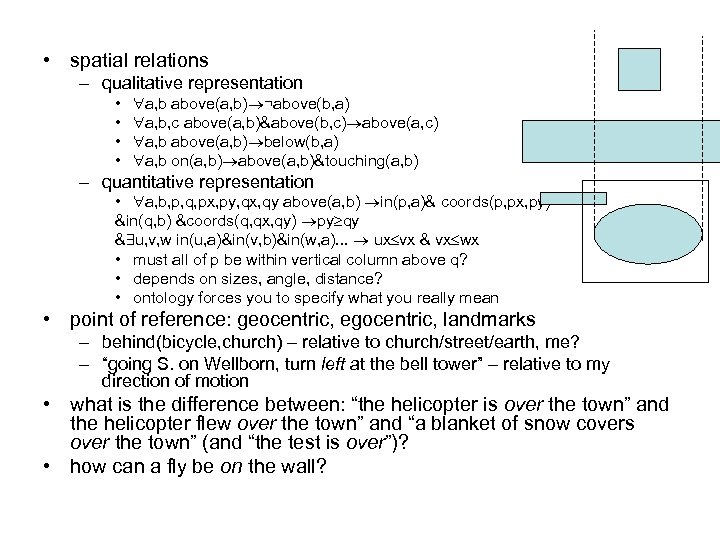• spatial relations – qualitative representation • • a, b above(a, b) ¬above(b, a) a, b, c above(a, b)&above(b, c) above(a, c) a, b above(a, b) below(b, a) a, b on(a, b) above(a, b)&touching(a, b) – quantitative representation • a, b, p, q, px, py, qx, qy above(a, b) in(p, a)& coords(p, px, py) &in(q, b) &coords(q, qx, qy) py qy & u, v, w in(u, a)&in(v, b)&in(w, a). . . ux vx & vx wx • must all of p be within vertical column above q? • depends on sizes, angle, distance? • ontology forces you to specify what you really mean • point of reference: geocentric, egocentric, landmarks – behind(bicycle, church) – relative to church/street/earth, me? – “going S. on Wellborn, turn left at the bell tower” – relative to my direction of motion • what is the difference between: “the helicopter is over the town” and the helicopter flew over the town” and “a blanket of snow covers over the town” (and “the test is over”)? • how can a fly be on the wall?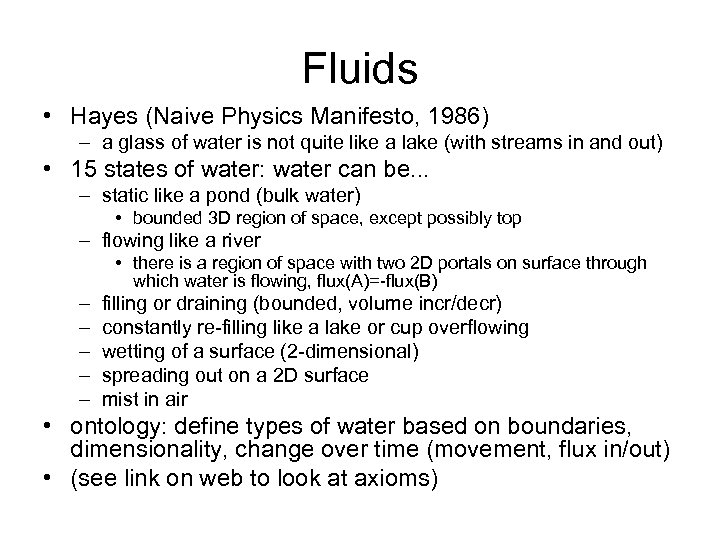Fluids • Hayes (Naive Physics Manifesto, 1986) – a glass of water is not quite like a lake (with streams in and out) • 15 states of water: water can be. . . – static like a pond (bulk water) • bounded 3 D region of space, except possibly top – flowing like a river • there is a region of space with two 2 D portals on surface through which water is flowing, flux(A)=-flux(B) – – – filling or draining (bounded, volume incr/decr) constantly re-filling like a lake or cup overflowing wetting of a surface (2 -dimensional) spreading out on a 2 D surface mist in air • ontology: define types of water based on boundaries, dimensionality, change over time (movement, flux in/out) • (see link on web to look at axioms)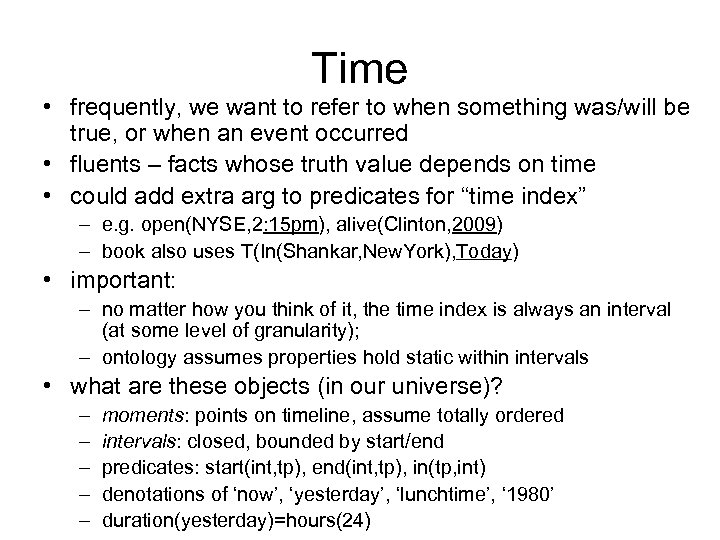Time • frequently, we want to refer to when something was/will be true, or when an event occurred • fluents – facts whose truth value depends on time • could add extra arg to predicates for “time index” – e. g. open(NYSE, 2: 15 pm), alive(Clinton, 2009) – book also uses T(In(Shankar, New. York), Today) • important: – no matter how you think of it, the time index is always an interval (at some level of granularity); – ontology assumes properties hold static within intervals • what are these objects (in our universe)? – – – moments: points on timeline, assume totally ordered intervals: closed, bounded by start/end predicates: start(int, tp), end(int, tp), in(tp, int) denotations of ‘now’, ‘yesterday’, ‘lunchtime’, ‘ 1980’ duration(yesterday)=hours(24)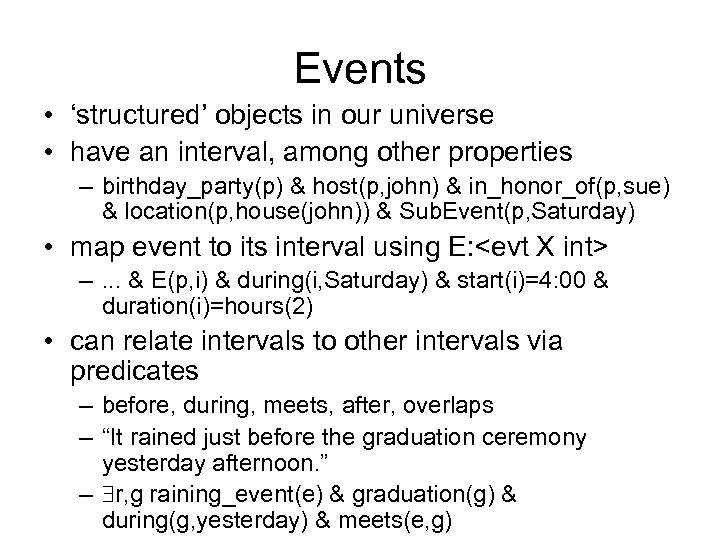Events • ‘structured’ objects in our universe • have an interval, among other properties – birthday_party(p) & host(p, john) & in_honor_of(p, sue) & location(p, house(john)) & Sub. Event(p, Saturday) • map event to its interval using E: –. . . & E(p, i) & during(i, Saturday) & start(i)=4: 00 & duration(i)=hours(2) • can relate intervals to other intervals via predicates – before, during, meets, after, overlaps – “It rained just before the graduation ceremony yesterday afternoon. ” – r, g raining_event(e) & graduation(g) & during(g, yesterday) & meets(e, g)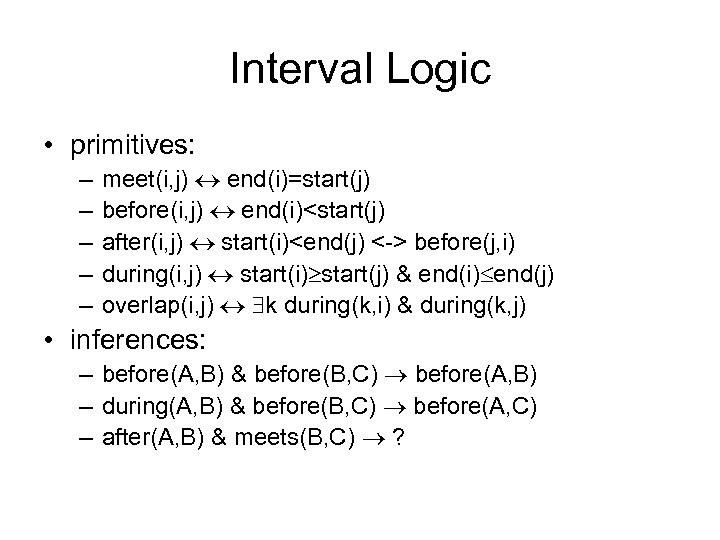Interval Logic • primitives: – – – meet(i, j) end(i)=start(j) before(i, j) end(i) before(j, i) during(i, j) start(i) start(j) & end(i) end(j) overlap(i, j) k during(k, i) & during(k, j) • inferences: – before(A, B) & before(B, C) before(A, B) – during(A, B) & before(B, C) before(A, C) – after(A, B) & meets(B, C) ?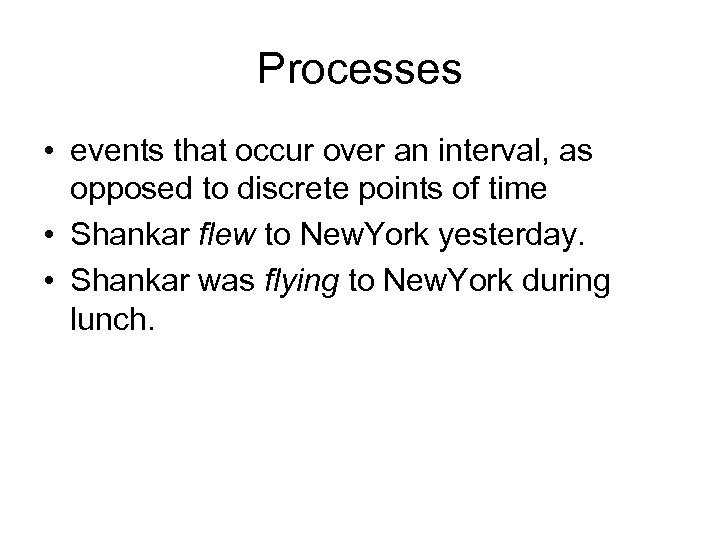Processes • events that occur over an interval, as opposed to discrete points of time • Shankar flew to New. York yesterday. • Shankar was flying to New. York during lunch.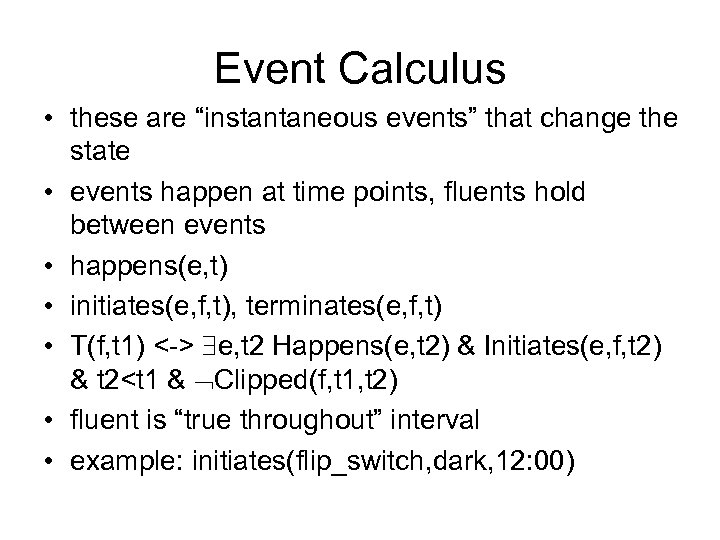Event Calculus • these are “instantaneous events” that change the state • events happen at time points, fluents hold between events • happens(e, t) • initiates(e, f, t), terminates(e, f, t) • T(f, t 1) <-> e, t 2 Happens(e, t 2) & Initiates(e, f, t 2) & t 2